#### Combining translation and rotation

Suppose a rotation by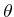is performed, followed by a translation by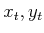. This can be used to place the robot in any desired position and orientation. Note that translations and rotations do not commute! If the operations are applied successively, each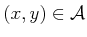is transformed to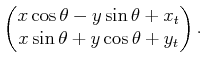(3.33)

The following matrix multiplication yields the same result for the first two vector components: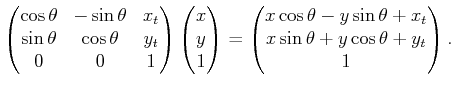(3.34)

This implies that the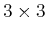matrix,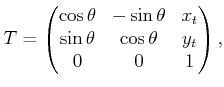(3.35)

represents a rotation followed by a translation. The matrix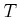will be referred to as a homogeneous transformation matrix. It is important to remember thatrepresents a rotation followed by a translation (not the other way around). Each primitive can be transformed using the inverse of, resulting in a transformed solid model of the robot. The transformed robot is denoted by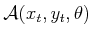, and in this case there are three degrees of freedom. The homogeneous transformation matrix is a convenient representation of the combined transformations; therefore, it is frequently used in robotics, mechanics, computer graphics, and elsewhere. It is called homogeneous because over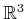it is just a linear transformation without any translation. The trick of increasing the dimension by one to absorb the translational part is common in projective geometry .

Steven M LaValle 2020-08-14